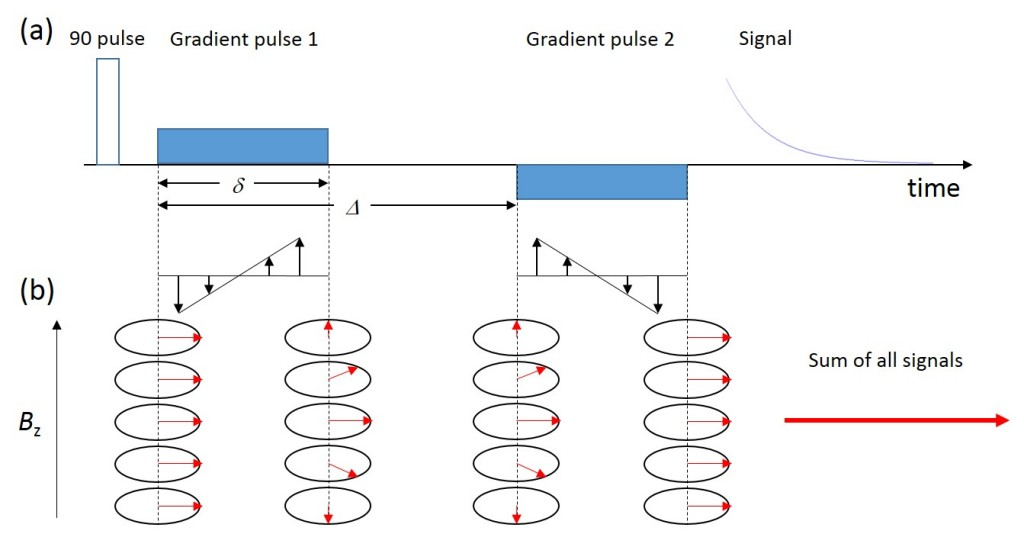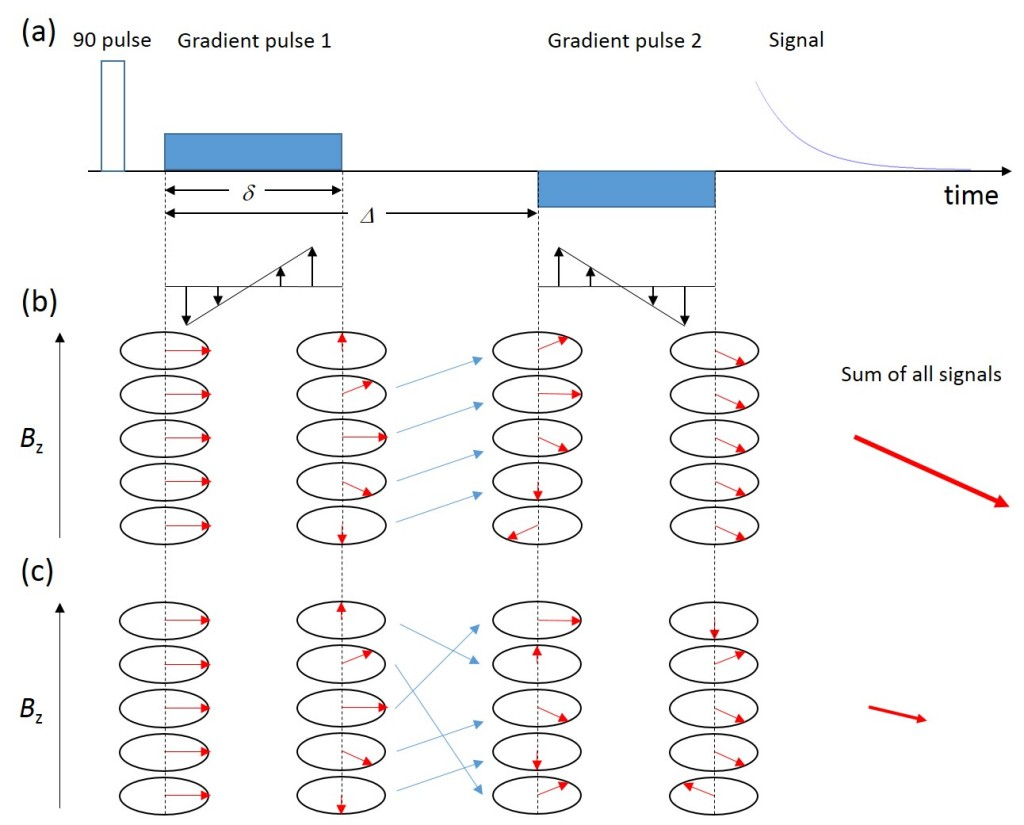# Gradients in NMR Spectroscopy – Part 3: Encoding for Displacement using Pairs of Pulsed Gradients

Part 2 of this series discussed how pulsed magnetic field gradients (PFGs) can be used to encode spins for their position, whilst still having the advantage of uniform field during signal acquisition.In this post we will see how pairs of pulsed gradients can encode for molecular displacement.

Consider the situation where we apply a second PFG after a time Δ. This second pulse is applied for exactly as long as the first pulse (let’s call the gradient pulse duration δ) with the same amplitude, but the direction is reversed. This is shown schematically in Figure 1 (a).

The evolution of the spin phases is shown in Figure 1 (b). Until the start of the second gradient pulse everything is the same as described in post number 2 on pulsed gradients. The second gradient pulse completely reverses the phase encoding created by the first one, so at the start of the acquisition all spins are in phase again. The measure signal is the sum coming from all the spins in the sample volume and is completely phased at the end of the second gradient pulse. As this is similar behaviour to the more commonly known spin echo, this sort of refocussing by gradient pulses is called a gradient echo.Figure 1: (a) Schematic diagram of a pulse sequence with a positive/negative pair of gradient pulses matched in amplitude and length.(b) The phase evolution of the spins at different locations along the gradient direction. Note that the gradient echo refocuses all phases at the end of the second gradient pulse.

At first glance it might not make sense to run such an experiment, where we encode spins for their location, only to undo it again. However, it does if the spins change their position between the two pulses. Let’s suppose that the time Δ is much longer than δ and that the spins are flowing all with the same speed along the direction of the gradient. At the end of the first gradient pulse, each spin carries a phase depending on the location where they were at the moment when the pulse was applied. During the time between the two gradient pulses, no extra phase is acquired, but they carry the phase information to the new position. Figure 2 (b) shows as an example the situation where each spin moves one position up. The second gradient pulse now unwinds the phase information according to the new position. Since the decoding and encoding phases don’t match any more due to the movement, each spin will end up with the same net phase shift at the end of the second gradient pulse. The measured signal, which is the sum coming from all the spins in the sample volume, is then the same amplitude as in Figure 1, but with a phase shift proportional to the distance that the spins travelled between the two gradient pulses.Figure 2: (a) Schematic diagram of a pulse sequence with a positive/negative pair of gradient pulses matched in amplitude and length.(b) The phase evolution of the spins in the case of coherent movement along the gradient direction. At the end of the second gradient pulse, all spins are in phase with each other, but there is a net phase shift proportional to the distance travelled. (c) The phase evolution of the spins in the case of random jumps along the gradient direction. At the end of the second gradient pulse, phases are distributed randomly, leading to a signal attenuation.

Finally, let’s consider the situation where the spins don’t move coherently, but jump randomly from one location to another. This type of movement is very important in science and will be addressed in the next post of this series.

Figure 2 (c) shows what happens to the spin phases if they randomly jump in position between the two gradient pulses. The nice helix-like phase encoding which we have at the end of the first gradient pulse is completely destroyed at the start of the second gradient pulse. At the end of the second gradient pulse, the spin phases are more or less randomly distributed. There will be spins with opposite phases cancelling each other out, so the measured signal will now be smaller than in the case of coherent motion.

As a summary of this post we conclude:

• A pair of matched gradient pulses encodes the spins for displacement.
• Coherent motion preserves the signal amplitude, but leads to a phase shift.
• Random motion results in an attenuation of the signal amplitude.

Please keep tuned for the next post in this series where we will look in more detail at random molecular jumps called diffusion.